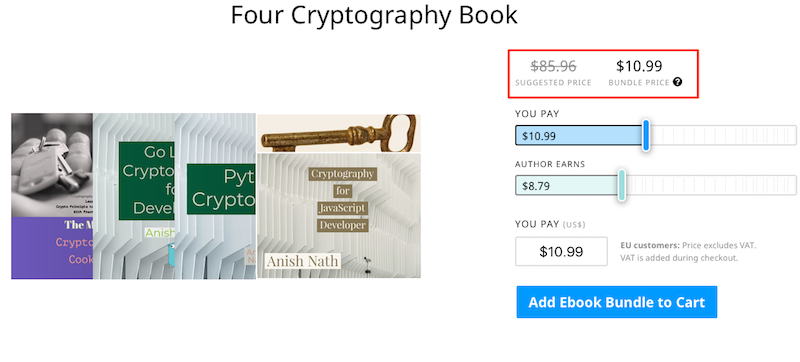# Web Crypto API ECDH generateKey/deriveBits and perform AES encryption and decryption

by Anish

Posted on Monday september 24, 2018

This sample chapter extracted from the book, Cryptography for JavaScript Developers.

The Web crypto api describes using Elliptic Curve Diffie-Hellman (ECDH) for key generation and key agreement, as specified by RFC6090.

The recognized algorithm name for this algorithm is "ECDH".

1. generateKey- Params: EcKeyGenParams KeyPair (Normalized Algorithm is "P-256", "P-384" or "P-521")
2. deriveBits Params: EcdhKeyDeriveParams Octet string (normalizedAlgorithm is "P-256", "P-384" or "P-521"))
3. importKey EcKeyImportParams Key (spki,jwk,raw,pkcs8)
4. exportKey None ArrayBuffer

Named Curve reference

1. secp256r1=P-256
2. secp384r1=P-384
3. secp521r1=P-521

## ECDH Demo

Named Curve
ECDH Derived Bits (Hex)
ECDH Derived Key Master (Hex)
ECDH Derived Key Usage (JWK)
ECDH Public Key (JWK) :
ECDH Private Key (JWK):
Encrypt Using ECDH Derived Key (Master) Output

This is the web cryptography api example of performing ECDH generateKey and derivebits, and then using generate key to encrypt and decrypt the message in AES

## ECDH Javascript example of using webcrypto api

Generate ECDH Keys

``````   window.crypto.subtle.generateKey({
name: "ECDH",
namedCurve: curve, //can be "P-256", "P-384", or "P-521"
},
true, //whether the key is extractable (i.e. can be used in exportKey)
["deriveKey", "deriveBits"] //can be any combination of "deriveKey" and "deriveBits"
)
.then(function(key) {
publicKey = key.publicKey;
privateKey = key.privateKey;
// For Demo Purpos Only Exported in JWK format
``````

ECDH exportKey Example (for demo purpose)

``````        window.crypto.subtle.exportKey("jwk", key.publicKey).then(
function(keydata) {
publicKeyhold = keydata;
publicKeyJson = JSON.stringify(publicKeyhold);
document.getElementById("ecdhpublic").value = publicKeyJson;
}
);

window.crypto.subtle.exportKey("jwk", key.privateKey).then(
function(keydata) {
privateKeyhold = keydata;
privateKeyJson = JSON.stringify(privateKeyhold);
document.getElementById("ecdhprivate").value = privateKeyJson;
}
);
``````

ECDH Derive key using name curved algorithms

``````        window.crypto.subtle.deriveKey({
name: "ECDH",
namedCurve: curve, //can be "P-256", "P-384", or "P-521"
public: publicKey, //an ECDH public key from generateKey or importKey
},
privateKey, //your ECDH private key from generateKey or importKey
{ //the key type you want to create based on the derived bits
name: "AES-CTR", //can be any AES algorithm ("AES-CTR", "AES-CBC", "AES-CMAC", "AES-GCM", "AES-CFB", "AES-KW", "ECDH", "DH", or "HMAC")
//the generateKey parameters for that type of algorithm
length: 256, //can be  128, 192, or 256
},

true, //whether the derived key is extractable (i.e. can be used in exportKey)
["encrypt", "decrypt"] //limited to the options in that algorithm's importKey
)
.then(function(keydata) {
//returns the exported key data

// For Demo Purpos Only Exported in JWK format
window.crypto.subtle.exportKey("jwk", keydata).then(
function(keydata) {
dKey = keydata;
document.getElementById("deriveKey").value = dKey.k
document.getElementById("deriveKeyUsage").value = JSON.stringify(dKey)
}
);
})
.catch(function(err) {
console.error(err);
});
``````

ECDH deriveBits

``````        window.crypto.subtle.deriveBits({
name: "ECDH",
namedCurve: curve, //can be "P-256", "P-384", or "P-521"
public: publicKey, //an ECDH public key from generateKey or importKey
},
privateKey, //from generateKey or importKey above
256 //the number of bits you want to derive
)
.then(function(bits) {
//returns an ArrayBuffer containing the signature
document.getElementById("cipherText").value = bytesToHexString(bits);
})
.catch(function(err) {
console.error(err);
});
})
.catch(function(err) {
console.error(err);
});
``````

}

Performing AES Encryption using ECDH generate Key, by importing JWK key and then deriving the AES encryption key and then encrypting the message, This snippet uses AES-CTR mode

``````obj = JSON.parse(deriveKeyUsage);
alg1 = obj.alg;
ext1 = obj.ext;
k1 = obj.k;
kty1 = obj.kty;

crypto.subtle.importKey("jwk", {
alg: alg1,
ext: ext1,
k: k1,
kty: kty1
}, "aes-ctr", false, ["encrypt"]).then(function(key) {
return crypto.subtle.encrypt({
name: "aes-ctr",
counter: iv,
length: 128
}, key, asciiToUint8Array(plainText));
}, failAndLog).then(function(cipherText) {
document.getElementById("cipherTextGCM").value = bytesToHexString(cipherText);
}, failAndLog);
``````

Performing AES Decryption using ECDH Derived Key, by importing JWK key and deriving the AES encryption key and then decryption the message, This snippet uses AES-CTR mode

``````obj = JSON.parse(deriveKeyUsage);
alg1 = obj.alg;
ext1 = obj.ext;
k1 = obj.k;
kty1 = obj.kty;

crypto.subtle.importKey("jwk", {
alg: alg1,
ext: ext1,
k: k1,
kty: kty1
}, "aes-ctr", false, ["decrypt"]).then(function(key) {
return crypto.subtle.decrypt({
name: "aes-ctr",
counter: iv,
length: 128
}, key, hexStringToUint8Array(plainText));
}, failAndLog).then(function(cipherText) {
}, failAndLog);``````

Next Reading Web Crypto API ECDSA Generate Keys Sign & verify Message

Thanku for reading !!! Give a Share for Support

Asking for donation sound bad to me, so i'm raising fund from The Modern Cryptography CookBook for Just \$9. Leanpub books Discount coupon first 100 reader. No hurry read the sample chapters here then decide.

Alternatively to support you can buy My all four Cryptography book Just \$10.99• The Modern Cryptography Book.
• Go lang Cryptography for developers
• Python Cryptography
• Cryptography for JavaScript Developer

Here